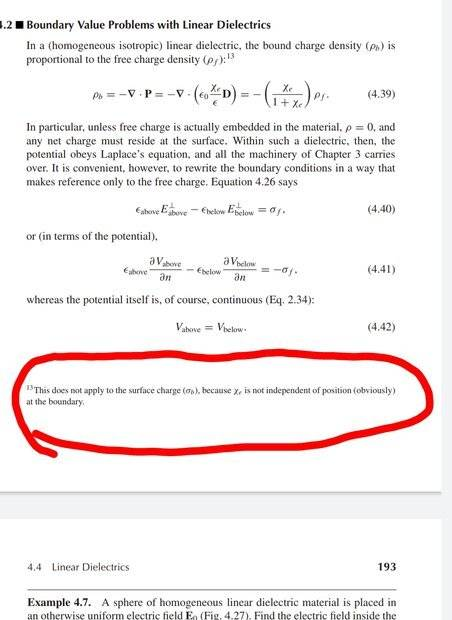# How could electric susceptibilbility depend on position?

• I
• Ahmed1029
Ahmed1029
In the statement encircled, what does Griffiths actually mean?•Delta2

Gold Member
2022 Award
It's a bit "nebulous". I guess what he considers is the effective (bound) surface charge of a homogeneous and isotropic dielectric.

[edit: corrected in view of #3]
The bound-charge density within the dielectric is
$$\rho=-\vec{\nabla} \cdot \vec{P},$$
which is 0, for ##\vec{P}=\text{const}##, within the dielectric. Trivially it's also 0 outside the dielectric, where is vacuum, i.e., no charges at all.

At the surface you have, however bound-surface charges, which you get by taking the "surface divergence". Let ##\vec{n}## be the surface-normal unity vector pointing out of the material. Then with a Gaussian pillbox with two sides parallel to the boundary of the dielectric, you get
$$\sigma=-\mathrm{Div} \vec{P}=\vec{n} \cdot \vec{P}.$$

Last edited:
•Delta2
Homework Helper
Gold Member
which is, for P→=0, within the dielectric
You probably mean ##\vec{P}=\text{constant}## inside the dielectric because if it was zero then ##\vec{D}=\epsilon_0\vec{E}+\vec{P}=\epsilon_0\vec{E}## inside the dielectric which doesn't look right...

•vanhees71
Homework Helper
At the boundary the susceptibility has different values on the two sides of the boundary.

•Ahmed1029 and vanhees71